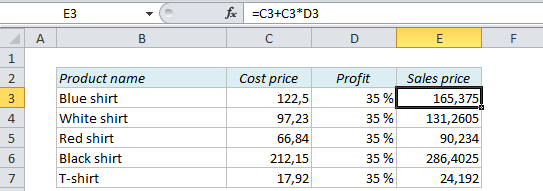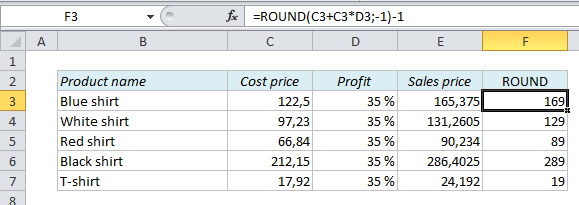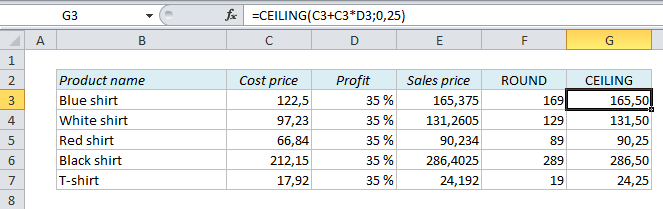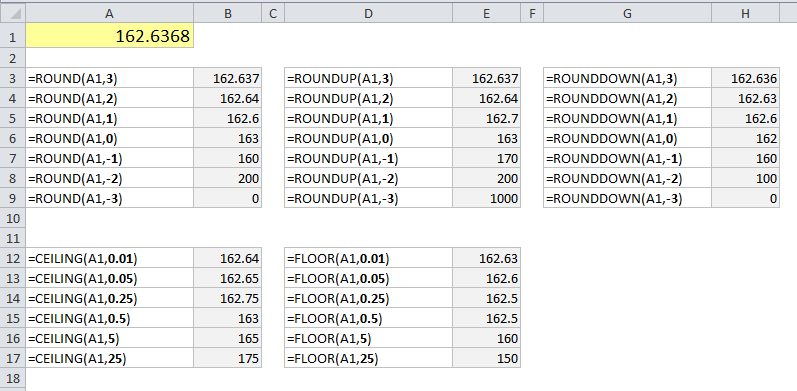# How to calculate prices and make them end with a certain number in Excel

When you calculate prices you usually know the cost price and the desired profit percentage, so all you have to do is to add the profit to the cost price in order to calculate the sales price. However, the price list might not look as nice as you want:If you want the prices to end with 9, 99, or .99, you can use the ROUND function. In E3, instead of =C3+C3*D3, we would use =ROUND(C3+C3*D3,-1)-1. The negative one (-1) inside the ROUND function means that Excel rounds up or down to the nearest power of ten, from 165.375 to 170, and the negative one in the end subtracts one from the result:
170 – 1 = 169.
We could also use ROUNDUP or ROUNDDOWN – they work in the same way as ROUND.But what of you want all your prices rounded up to the nearest quarter or nickel? The ROUND functions can only round up or down to different multiples of 10: .1, 1, 10, 100 etc., and now we want a multiple of .05 or .25. We have to use the CEILING function: =CEILING(C3+C3*D3,0.25). The last value in the formula is the multiple you want to round up to, so if you replace 0.25 with 0.05 it will round up to the nearest nickel.## Summary

Take a look at the table below to see how the numbers change with ROUND, ROUNDUP, ROUNDDOWN, CEILING, and FLOOR## More Excel tutorials:

Are you using a non-English version of Excel? Click here for translations of the 100 most common functions.